GeeksforGeeks App
Open AppBrowser
Continue

# Count frequency of itemsets in Pandas DataFrame

In this article, we are going to explain how we can Count the frequency of itemsets in the given DataFrame in Pandas. Using the count(), size() method, Series.value_counts(), and pandas.Index.value_counts() method we can count the number of frequency of itemsets in the given DataFrame. Here, we are going to explain several examples of how to use these functions in practice.

Raw Data: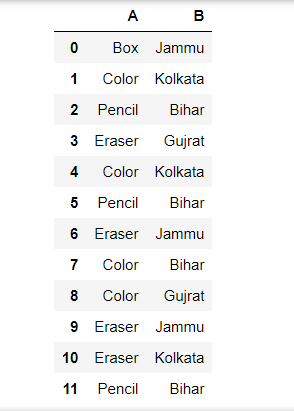Example 1:

Using Series.value_counts(): This method is applicable to pandas.Series object. Since each DataFrame object is a collection of Series objects, we can apply this method to get the frequency counts of values in one column.

## Python3

 `import` `pandas as pd` `df ``=` `pd.DataFrame({``    ``'A'``: [``'Box'``, ``'Color'``, ``'Pencil'``, ``'Eraser'``,``          ``'Color'``, ``'Pencil'``, ``'Eraser'``, ``'Color'``,``          ``'Color'``, ``'Eraser'``, ``'Eraser'``, ``'Pencil'``],` `    ``'B'``: [``'Jammu'``, ``'Kolkata'``, ``'Bihar'``, ``'Gujarat'``,``          ``'Kolkata'``, ``'Bihar'``, ``'Jammu'``, ``'Bihar'``,``          ``'Gujarat'``, ``'Jammu'``, ``'Kolkata'``, ``'Bihar'``]``})` `count ``=` `df[``'A'``].value_counts()``display(count)` `count ``=` `df[``'B'``].value_counts()``display(count)`

Output: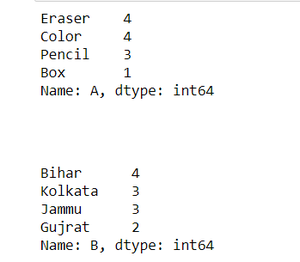Example 2: Using Pandas dataframe.size()

It returns a total number of elements, it is compared by multiplying rows and columns returned by the shape method.

## Python3

 `import` `pandas as pd` `df ``=` `pd.DataFrame({``    ``'A'``: [``'Box'``, ``'Color'``, ``'Pencil'``, ``'Eraser'``,``          ``'Color'``, ``'Pencil'``, ``'Eraser'``, ``'Color'``,``          ``'Color'``, ``'Eraser'``, ``'Eraser'``, ``'Pencil'``],` `    ``'B'``: [``'Jammu'``, ``'Kolkata'``, ``'Bihar'``, ``'Gujarat'``,``          ``'Kolkata'``, ``'Bihar'``, ``'Jammu'``, ``'Bihar'``,``          ``'Gujarat'``, ``'Jammu'``, ``'Kolkata'``, ``'Bihar'``]``})` `freq ``=` `df.groupby([``'A'``]).size()``display(freq)` `freq ``=` `df.groupby([``'B'``]).size()``display(freq)`

Output: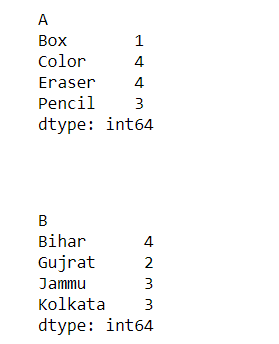Example 3: Using Pandas reset_index()

It is a method to reset the index of a Data Frame. reset_index() method sets a list of integers ranging from 0 to length of data as an index.

## Python3

 `import` `pandas as pd` `df ``=` `pd.DataFrame({``    ``'A'``: [``'Box'``, ``'Color'``, ``'Pencil'``, ``'Eraser'``,``          ``'Color'``, ``'Pencil'``, ``'Eraser'``, ``'Color'``,``          ``'Color'``, ``'Eraser'``, ``'Eraser'``, ``'Pencil'``],` `    ``'B'``: [``'Jammu'``, ``'Kolkata'``, ``'Bihar'``, ``'Gujarat'``,``          ``'Kolkata'``, ``'Bihar'``, ``'Jammu'``, ``'Bihar'``,``          ``'Gujarat'``, ``'Jammu'``, ``'Kolkata'``, ``'Bihar'``]``})`  `freq ``=` `df.groupby([``'A'``])[``'B'``].agg(``'count'``).reset_index()``display(freq)` `freq ``=` `df.groupby([``'B'``])[``'A'``].agg(``'count'``).reset_index()``display(freq)`

Output: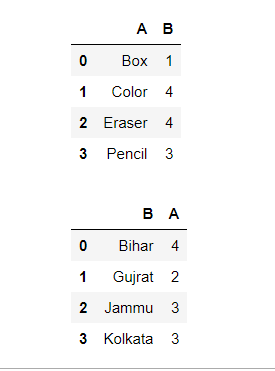Example 4: Using Pandas dataframe.count()

It is used to count the no. of non-NA/null observations across the given axis. It works with non-floating type data as well.

## Python3

 `import` `pandas as pd` `df ``=` `pd.DataFrame({``    ``'A'``: [``'Box'``, ``'Color'``, ``'Pencil'``, ``'Eraser'``,``          ``'Color'``, ``'Pencil'``, ``'Eraser'``, ``'Color'``,``          ``'Color'``, ``'Eraser'``, ``'Eraser'``, ``'Pencil'``],` `    ``'B'``: [``'Jammu'``, ``'Kolkata'``, ``'Bihar'``, ``'Gujarat'``,``          ``'Kolkata'``, ``'Bihar'``, ``'Jammu'``, ``'Bihar'``,``          ``'Gujarat'``, ``'Jammu'``, ``'Kolkata'``, ``'Bihar'``]``})` `freq ``=` `df.groupby([``'A'``]).count()``display(freq)` `freq ``=` `df.groupby([``'B'``]).count()``display(freq)`

Output: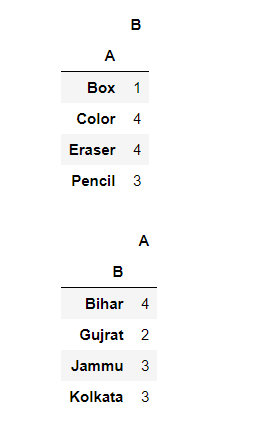My Personal Notes arrow_drop_up